# Lecture in Area Problem and the Riemann Sum Part 1

(Last Updated On: March 21, 2020)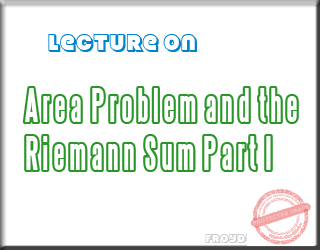To give you a brief review, remember that Calculus is a branch of mathematics that addressed two problems: finding instantaneous rate of change and the area between a curve and the x-axis. In differential calculus you have learned that the derivative gives the instantaneous rate of change. It answers the question, How fast is “something” changing?. The antiderivative or integral gives the area between a curve and the x– axis. It answers the question, How much is the “total”?.
In my next lectures you are going to learn to compute the area under a curve and area between a curve using definite integration. I know you are excited to solve problems, but before that you must focus to learn this three-part series lectures first.

### Area of a Rectangle

For now, I am going to discuss the techniques for estimating the area under a curve. Let us consider a rectangle. How can we compute the area of rectangle with height h and length b – a ? Yes, it is very easy simply the product  h ⋅ (b − a).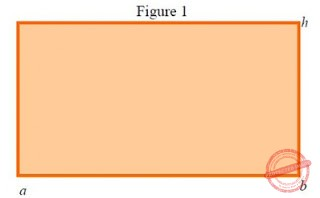### Area Under a Curved

We are going to assume a region that is bounded with a curved side, What we want to do is determine the area.  Consider, for example, the region shown below that is bounded by f, the x-axis, and the lines x = a and x = b .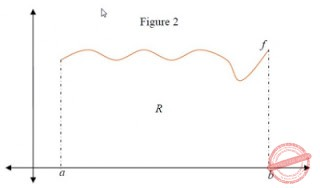From the first look, we can’t possibly imagine how to compute for the exact area of R. We can say that getting the area of the region between f and the x-axis is really a hard task to do.  This problems made our Mathematicians of long ago exerted a lot of effort to uncover the solutions for this problems.

### Enclosed the region R with a Rectangle

Enclosing a rectangle over R as in Figure 3 reveals a simple process for easy computing an estimate for the area.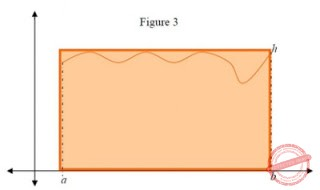Figure 3 shows that the product h ⋅ (b − a) gives a gross yet reasonable estimate for the area in R.  If we let A equals the area of R, then clearly we can say that  h ⋅ (b − a) > A since h is greater than or equal to all values of f along the interval [a,b] .

### Divide the Rectangle for Much Better Estimate

To obtain a much better estimate,  we divide the rectangle with height h into n rectangles of some uniform width equal to (b − a) /n with heights determined in some manner by f
In Figure 4 below, each rectangle intersects f at the top left corner so that the height of each rectangle is determined by f(xi ) where xi corresponds to the left end point of the subintervals of [a,b] that comprise the widths of the rectangles. The sum of the areas of the rectangles provides a much improved estimate of the area of R.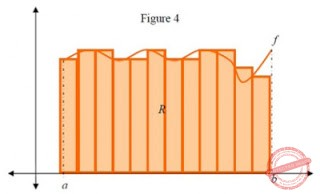The number of rectangles used to generate an estimate is arbitrary, but we can conclude that the greater the number of rectangles used the more accurate the estimate will be. Similarly, the choice to determine the height of each rectangle using f(xi ) where xi  corresponds to the left end point of the subintervals of [a,b] is also arbitrary. The points xi  could refer to the right end points of the subintervals of [a,b] , the midpoints of the subintervals of [a,b] , or even just any random point within each subinterval of [a,b] .

### Options in choosing the point xi or the height of the Rectangle

I just want to highlight or give emphasis to this crucial part of estimating the area because it is important for you to know what would be the difference if you choose the right end points, the left end points or the midpoints. To answer the question, I made a separate post because I don’t want this post to be very long. Just follow the link Area Problem and the Riemann Sum Part III and at the end of the post you will find a link to bring you back here.

### Welcome back

I suppose you already read and learned the different methods of estimating the area. Let’s continue from Figure 4, we are going to write an approximation formula for A, the area of R. Since the number of rectangles used for the estimate is arbitrary, let’s represent the number of rectangles with n. Recall that the rectangles span [a,b], and each rectangle has a width given by (b − a) /n . We will let x1, x2, . . ., xn be sample points taken from the first, second, . . ., and nth subintervals. Accordingly, we have the following.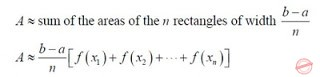The notation above is easy to evaluate if n let’s say five, but if n is large number, the notation becomes somewhat very long and complex. In that case, we are going to use sigma notation. The Greek letter sigma, Σ, indicates the summation of terms. Letting xi  be some arbitrary point within each closed subinterval of [a,b], and letting Δx represent the width of each rectangle, (b − a) /n , we
obtain the following.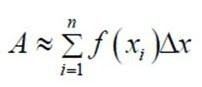The letter i is called the index of summation and stands for the ith (that is, the first, second, . . .,nth) subinterval. The sum is called a Riemann sum.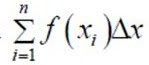I will let you absorb all these things first before we continue with the Riemann Sum. If you have any clarification just leave it on the comment section below. Now if you are ready you can proceed to Part II of the series.

credit: Randell Simpson (Temple College)©2013 www.PinoyBIX.com

P inoyBIX educates thousands of reviewers and students a day in preparation for their board examinations. Also provides professionals with materials for their lectures and practice exams. Help me go forward with the same spirit.

“Will you subscribe today via YOUTUBE?”

Subscribe

© 2014 PinoyBIX Engineering. © 2019 All Rights Reserved | How to Donate? |